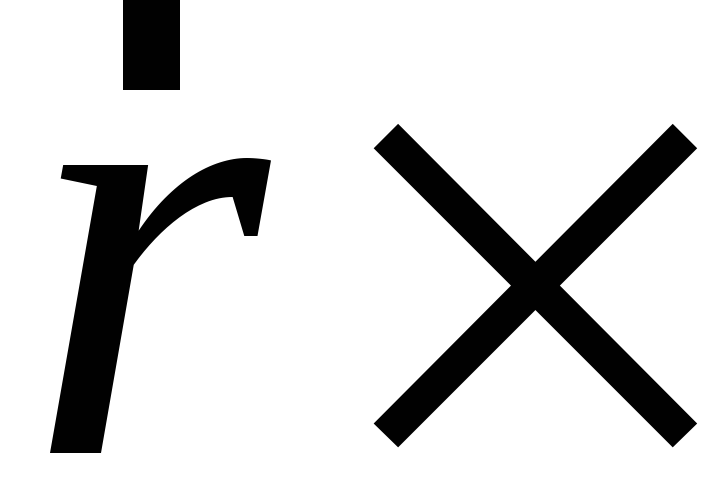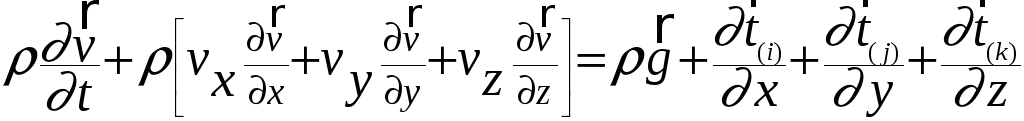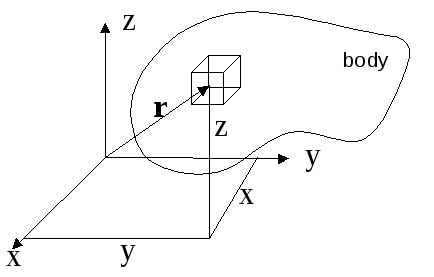# Conservation of Angular Momentum

  2001, W. E. Haisler Chapter 4: Conservation of Angular Momentum Conservation of Angular Momentum (chapter 4) Angular Momentum is defined as the moment of the Linear Momentum about some spatial reference point. Thus, it is the vector cross product with the linear momentum. angular momentum =linear momentum Like linear momentum, angular momentum is conserved. Thus, like conservation of linear momentum, we have the same spatial and time derivatives; except they operate on the angular momentum. Recall conservation of linear momentum for a differential system as shown below:Share with your friends: